Ex 9.2

Chapter 9 Class 11 Sequences and Series
Serial order wise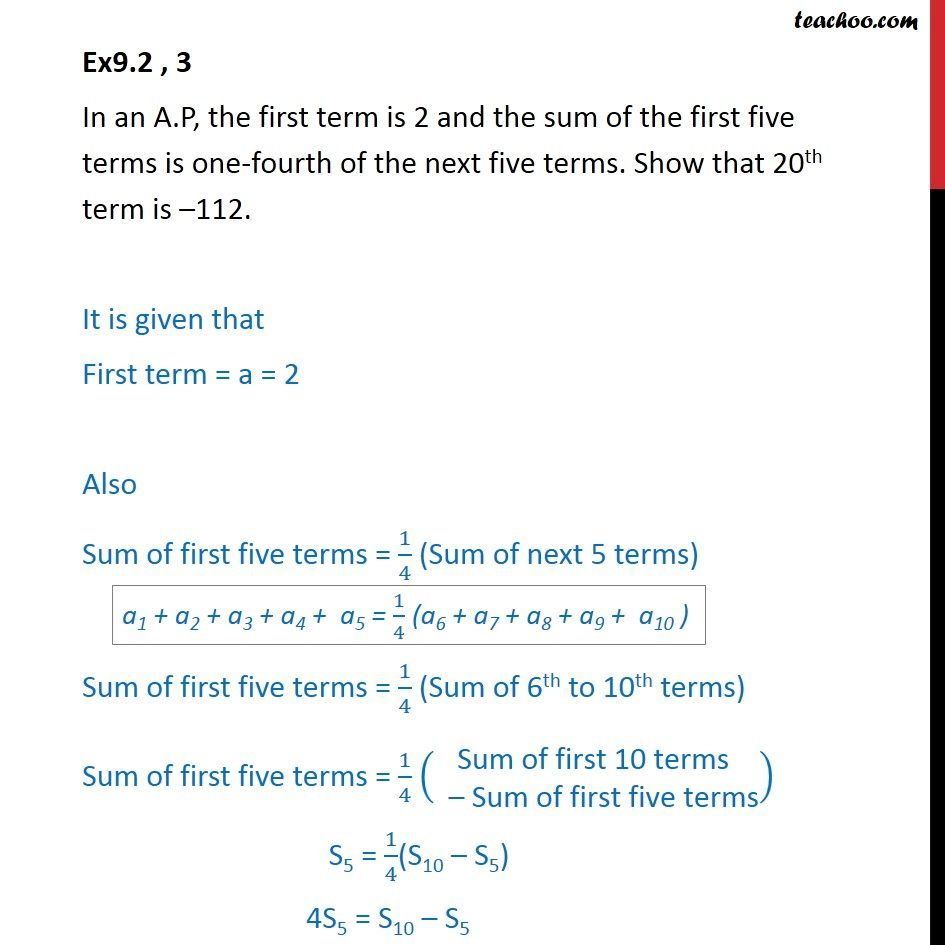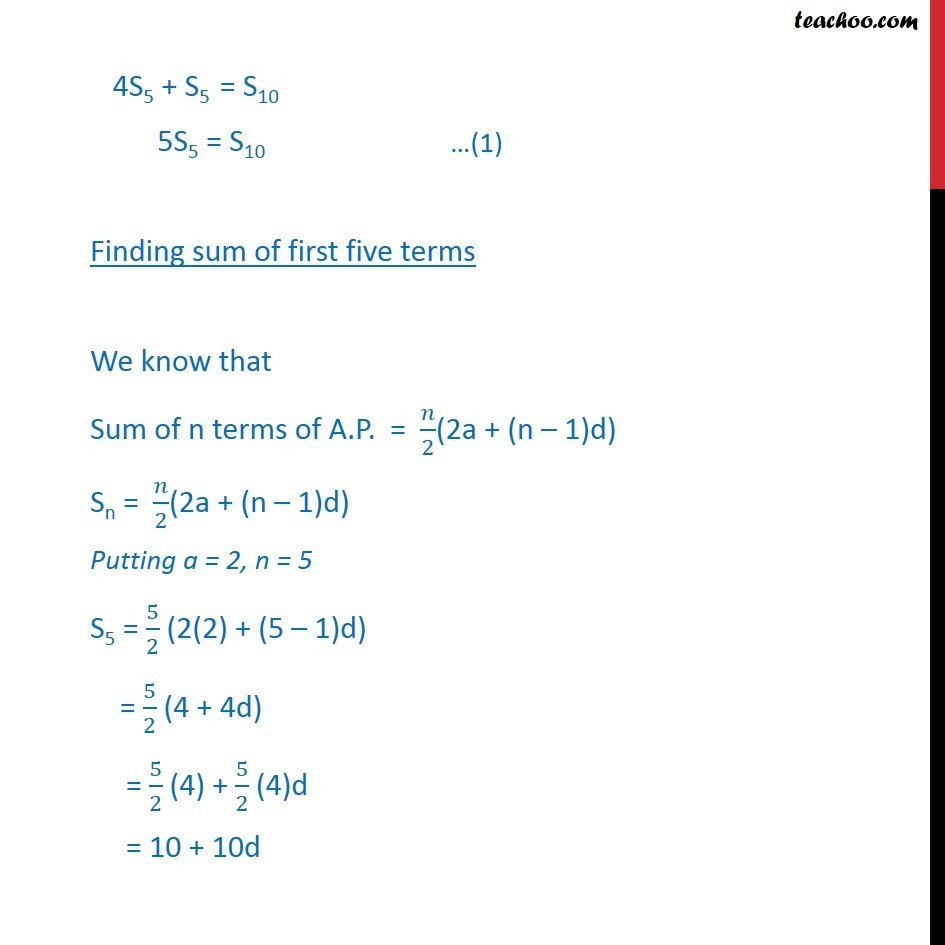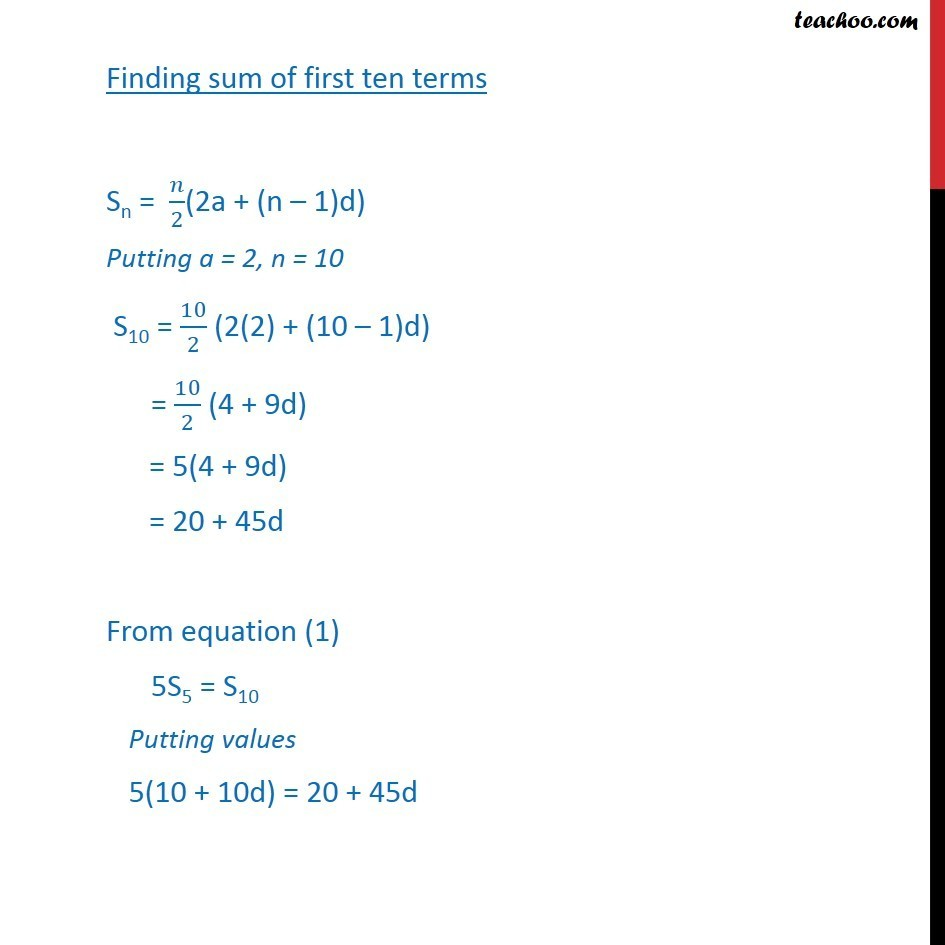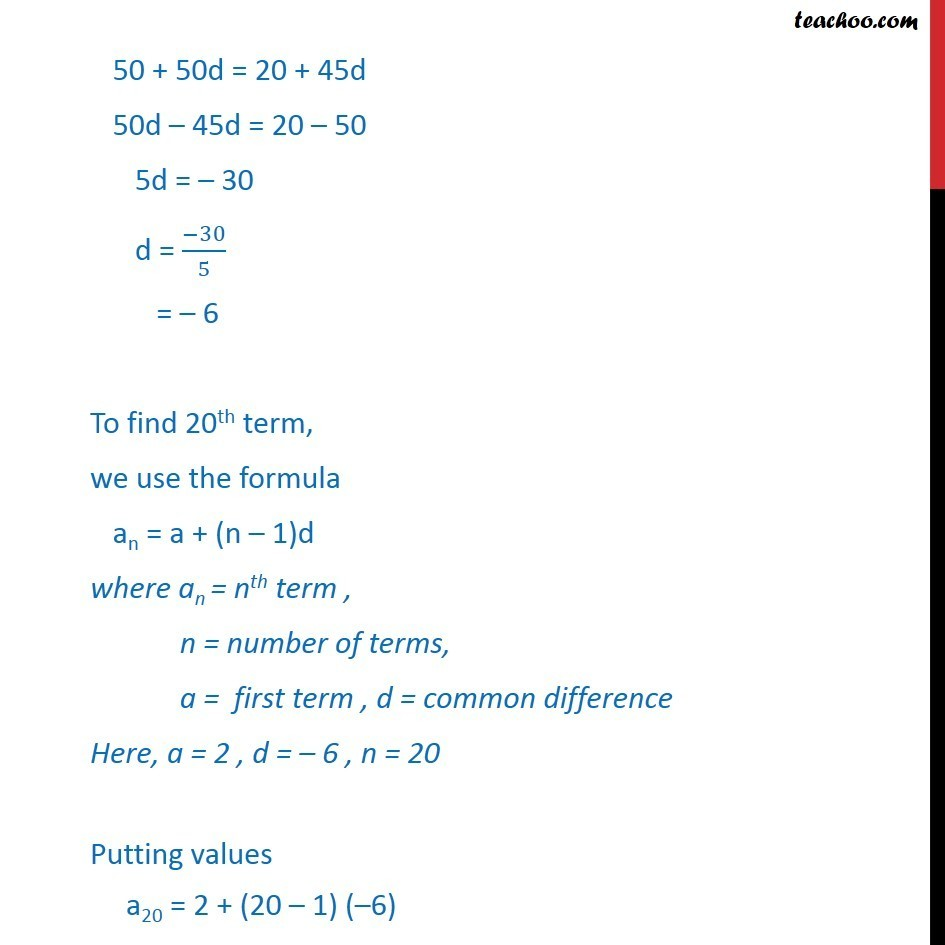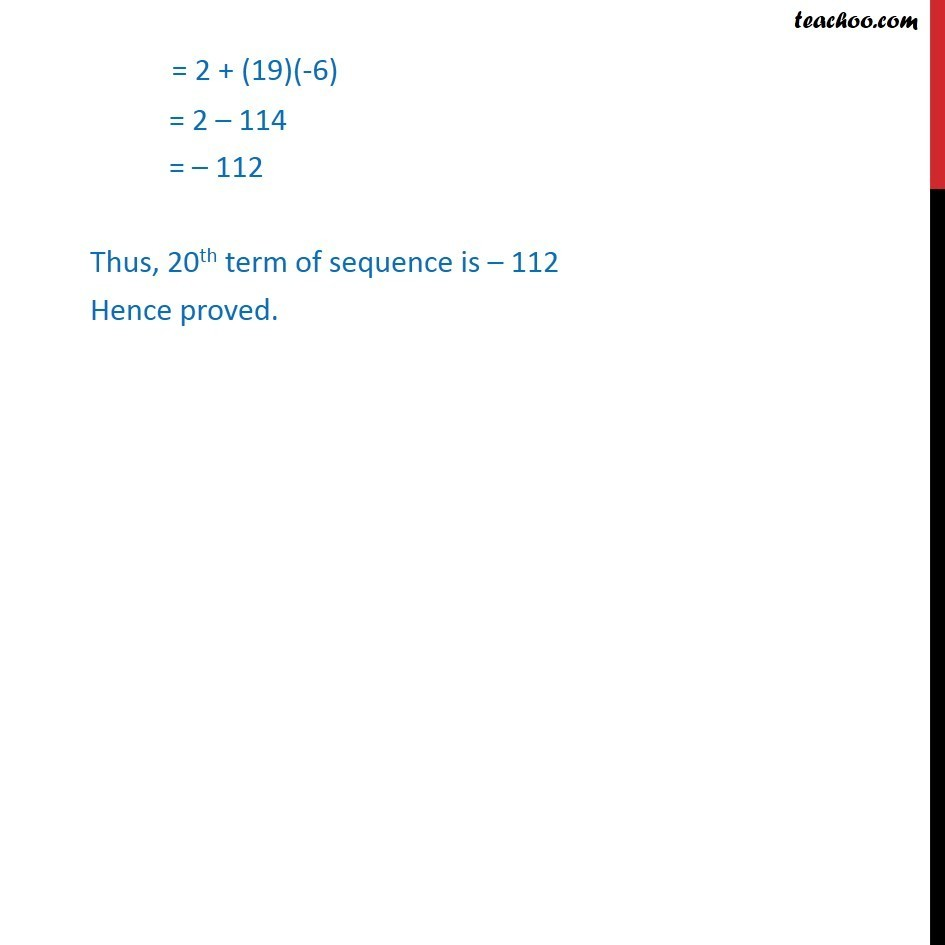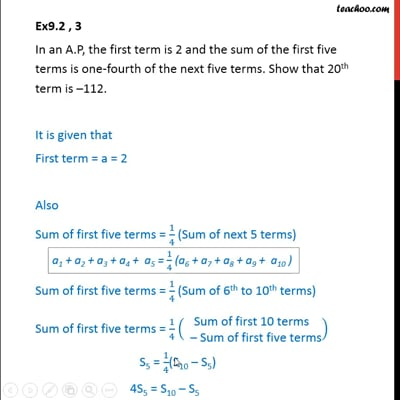This video is only available for Teachoo black users

Solve all your doubts with Teachoo Black (new monthly pack available now!)

### Transcript

Ex9.2 , 3 In an A.P, the first term is 2 and the sum of the first five terms is one-fourth of the next five terms. Show that 20th term is 112. It is given that First term = a = 2 Also Sum of first five terms = 1/4 (Sum of next 5 terms) Sum of first five terms = 1/4 (Sum of 6th to 10th terms) Sum of first five terms = 1/4 ( 8( ("Sum of first 10 terms " @" Sum of first five terms" ))) S5 = 1/4(S10 S5) 4S5 = S10 S5 4S5 + S5 = S10 5S5 = S10 Finding sum of first five terms We know that Sum of n terms of A.P. = /2(2a + (n 1)d) Sn = /2(2a + (n 1)d) Putting a = 2, n = 5 S5 = 5/2 (2(2) + (5 1)d) = 5/2 (4 + 4d) = 5/2 (4) + 5/2 (4)d = 10 + 10d Finding sum of first ten terms Sn = /2(2a + (n 1)d) Putting a = 2, n = 10 S10 = 10/2 (2(2) + (10 1)d) = 10/2 (4 + 9d) = 5(4 + 9d) = 20 + 45d From equation (1) 5S5 = S10 Putting values 5(10 + 10d) = 20 + 45d 50 + 50d = 20 + 45d 50d 45d = 20 50 5d = 30 d = ( 30)/5 = 6 To find 20th term, we use the formula an = a + (n 1)d where an = nth term , n = number of terms, a = first term , d = common difference Here, a = 2 , d = 6 , n = 20 Putting values a20 = 2 + (20 1) ( 6) = 2 + (19)(-6) = 2 114 = 112 Thus, 20th term of sequence is 112 Hence proved.